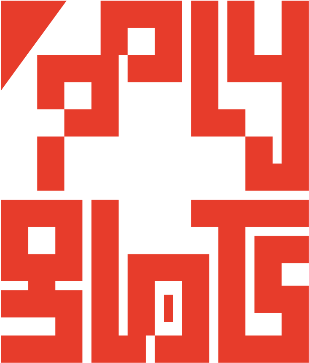# polygl0ts

### EPFL's CTF team# Insomnihack 2022: EmojiHell

## Description

In this challenge, we are provided with a Python program, along with the output it generated when passed the flag. To start, we break down the program to see what it does.

First, it seeds the RNG with a secret value and uses it to define a one-to-one mapping between the numbers `00-99` and the 100 emojis in its code alphabet.

``````perms = ...  # 100 distinct emojis
perms = perms.replace(" ","")[:100]
perms = ''.join(random.sample(perms,len(perms)))
``````

Second, it uses that mapping to create a simple substitution cipher where every pair of decimal digits in a number is replaced with an emoji. We will need to figure out that cipher to obtain the flag.

``````def int2Emoji(i):
return perms[i%100]

def bigInt2Emoji(i):
output = ""
s = str(i)
for i in range(0, len(s), 2):
output += int2Emoji(int(s[i:i+2]))
return output
``````

Third, it computes some values and shows them through the substitution cipher. More precisely, it goes through every number between 0 and 99 in a random order and prints the number, its square, its cube, its fourth and fifth powers, and its product with its predecessor.

``````for i in random.sample(range(100),100):
print(int2Emoji(i),int2Emoji(i**2),int2Emoji(i**3),int2Emoji(i**4),int2Emoji(i**5), int2Emoji((i-1)*i))
``````

Finally, it shows some values that are computed based on the flag.

``````intMsg = int.from_bytes(message.encode('utf-8'), byteorder='big')

a, b, c = 0, 0, 0

while a + b + c >= intMsg or a == 0:
a = random.randint(intMsg>>3, intMsg)
b = random.randint(intMsg>>3, intMsg)
c = random.randint(intMsg>>3, intMsg)
d = intMsg-a-b-c

print()
print(bigInt2Emoji(a))
print(bigInt2Emoji(b))
print(bigInt2Emoji(c))
print(bigInt2Emoji(d))
``````

## Solution

Since we know exactly which items the sample contains, but not in which order, we perform frequency analysis on the sample. If an emoji has a unique occurence count, we can match it with a number. This decodes 4 emojis out of 100.

Next, we use the structure of the sample to build pairs of emojis for which we know that second emoji maps to the square modulo 100 of the first. We do the same for cubes and other sampled relations. We then do the simple case: if the first item is already decoded, we directly compute the second item and infer that it is mapped to the emoji. We repeat that process until we can no longer find new decodes. We can crack 24 new emojis that way.

The next step is to try to go from the second element to the first. This is more challenging since in this algebra, an element can have multiple square roots (respectively cubic, etc.). To do so, we maintain a set of candidate values for each undecoded emoji that initially contain every emoji. Then we iterate through every relation and remove candidates that don’t satisfy the equation. If at the end of the process an emoji is left with a single candidate, this is a match. By doing this iteratively we get all but 10 emojis.

Finally, we go back in our solution to insert some manual guesses. The solution can automatically reject a guess if it leads to a contradiction. With just 2 guesses, we found the full substitution.

## Flag

`INS{😿😾🙀😹😺😸😻😼😀🤣😄😅😆😊😁😂}`

## Code

You can find the code for our solution here.